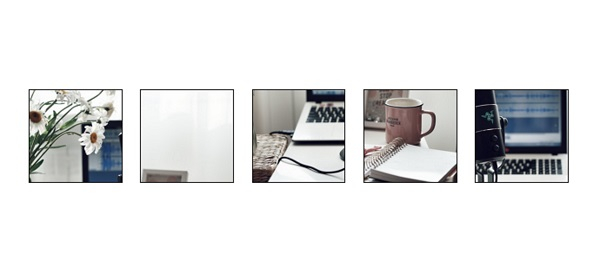# PyTorch â€“ FiveCrop Transformation

To crop a given image into four corners and the central crop, we apply FiveCrop() transformation. It's one of the transformations provided by the torchvision.transforms module. This module contains many important transformations that can be used to perform different types of manipulations on the image data.

FiveCrop() transformation accepts both PIL and tensor images. A tensor image is a torch Tensor with shape [C, H, W], where C is the number of channels, H is the image height, and W is the image width. If the image is neither a PIL image nor a tensor image, then we first convert it to a tensor image and then apply the FiveCrop transformation.

## Syntax

torchvision.transforms.FiveCrop(size)

where size is the desired crop size. size is a sequence like (h, w), where h and w are the height and width of each cropped image. If size is an int, the cropped images are square.

It returns a tuple of five cropped images, four corner and one central image.

## Steps

We could use the following steps to crop an image into four images and the central crop with given size −

• Import the required libraries. In all the following examples, the required Python libraries are torch, Pillow, and torchvision. Make sure you have already installed them.

import torch
import torchvision
import torchvision.transforms as transforms
from PIL import Image
• Read the input image. The input image is a PIL image or a torch tensor.

img = Image.open('recording.jpg')
• Define a transform to crop the image into four corners and the central crop. The crop size is set to (150, 300) for rectangular crop and 250 for square crop. Change the crop size according your need.

# transform for rectangular crop
transform = transforms.FiveCrop((200,250))

# transform for square crop
transform = transforms.FiveCrop(250)
• Apply the above-defined transform on the input image to crop the image into four corners and the central crop.

img = transform(img)
• Show all the five cropped images.

## Input Image

We will use this image in both the following examples.## Example 1

In below Python3 program we crop four corner and a central crop. The five cropped images are rectangular in shape.

# import required libraries
import torch
import torchvision.transforms as transforms
from PIL import Image
import matplotlib.pyplot as plt

img = Image.open('recording.jpg')

# define a transform to crop the image into four
# corners and the central crop
transform = transforms.FiveCrop((150, 300))

# apply the above transform on the image
imgs = transform(img)

# This transform returns a tuple of 5 images
print(type(imgs))
print("Total cropped images:",len(imgs))

## Output

<class 'tuple'>
Total cropped images: 5

## Example 2

In the following Python3 program, we crop fours corner and a central crop. The five cropped images are square in shape.

# import required libraries
import torch
import torchvision.transforms as transforms
from PIL import Image
import matplotlib.pyplot as plt

img = Image.open('recording.jpg')

# define a transform to crop the image into four
# corners and the central crop
transform = transforms.FiveCrop(200)

# apply the above transform on the image
imgs = transform(img)

# Define a figure of size (8, 8)
fig=plt.figure(figsize=(8, 8))

# Define row and cols in the figure
rows, cols = 1, 5

# Display all 5 cropped images
for j in range(0, cols*rows):
plt.show()